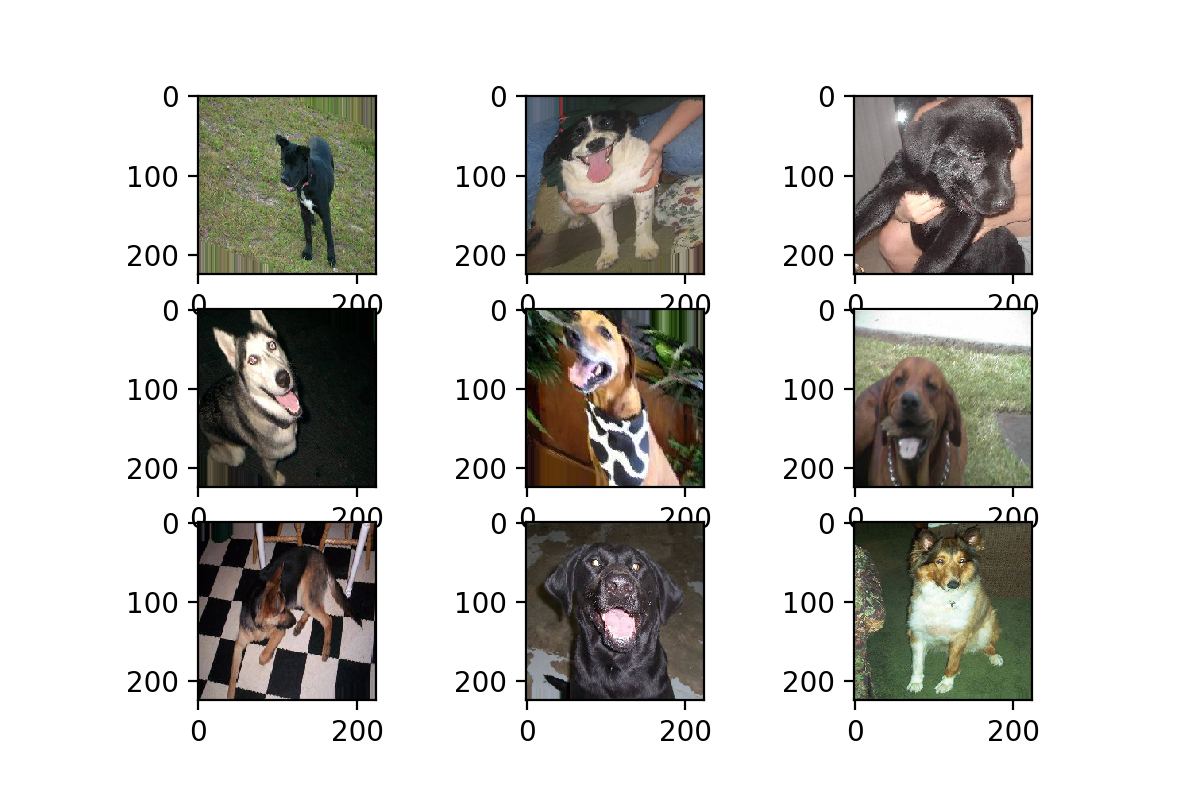``````keras.preprocessing.image.ImageDataGenerator(featurewise_center=False,
samplewise_center=False,
featurewise_std_normalization=False,
samplewise_std_normalization=False,
zca_whitening=False,
zca_epsilon=1e-6,
rotation_range=0.,
width_shift_range=0.,
height_shift_range=0.,
shear_range=0.,
zoom_range=0.,
channel_shift_range=0.,
fill_mode='nearest',
cval=0.,
horizontal_flip=False,
vertical_flip=False,
rescale=None,
preprocessing_function=None,
data_format=K.image_data_format())
``````

## 1. featurewise

``````datagen = image.ImageDataGenerator(featurewise_center=True,
featurewise_std_normalization=True)``````

featurewise_center的官方解释："Set input mean to 0 over the dataset, feature-wise." 大意为使数据集去中心化（使得其均值为0），而samplewise_std_normalization的官方解释是“ Divide inputs by std of the dataset, feature-wise.”，大意为将输入的每个样本除以其自身的标准差。这两个参数都是从数据集整体上对每张图片进行标准化处理，我们看看效果如何：

## 2. samplewise

``````datagen = image.ImageDataGenerator(samplewise_center=True,
samplewise_std_normalization=True)``````

samplewise_center的官方解释为：“ Set each sample mean to 0.”，使输入数据的每个样本均值为0；samplewise_std_normalization的官方解释为：“Divide each input by its std.”，将输入的每个样本除以其自身的标准差。这个月featurewise的处理不同，featurewise是从整个数据集的分布去考虑的，而samplewise只是针对自身图片，效果如图3：

## 3. zca_whtening

``datagen = image.ImageDataGenerator(zca_whitening=True)``

zca白化的作用是针对图片进行PCA降维操作，减少图片的冗余信息，保留最重要的特征，细节可参看：Whitening transformation--维基百科Whitening--斯坦福

## 4. rotation range

``datagen = image.ImageDataGenerator(rotation_range=30)``

rotation range的作用是用户指定旋转角度范围，其参数只需指定一个整数即可，但并不是固定以这个角度进行旋转，而是在 [0, 指定角度] 范围内进行随机角度旋转。效果如图5：

## 5. width_shift_range & height_shift_range

``datagen = image.ImageDataGenerator(width_shift_range=0.5,height_shift_range=0.5)``

width_shift_range & height_shift_range 分别是水平位置评议和上下位置平移，其参数可以是[0, 1]的浮点数，也可以大于1，其最大平移距离为图片长或宽的尺寸乘以参数，同样平移距离并不固定为最大平移距离，平移距离在 [0, 最大平移距离] 区间内。效果如图6：

## 6. shear_range

``datagen = image.ImageDataGenerator(shear_range=0.5)``

shear_range就是错切变换，效果就是让所有点的x坐标(或者y坐标)保持不变，而对应的y坐标(或者x坐标)则按比例发生平移，且平移的大小和该点到x轴(或y轴)的垂直距离成正比。

## 7. zoom_range

``datagen = image.ImageDataGenerator(zoom_range=0.5)``

zoom_range参数可以让图片在长或宽的方向进行放大，可以理解为某方向的resize，因此这个参数可以是一个数或者是一个list。当给出一个数时，图片同时在长宽两个方向进行同等程度的放缩操作；当给出一个list时，则代表[width_zoom_range, height_zoom_range]，即分别对长宽进行不同程度的放缩。而参数大于0小于1时，执行的是放大操作，当参数大于1时，执行的是缩小操作。

## 8. channel_shift_range

``datagen = image.ImageDataGenerator(channel_shift_range=10)``

channel_shift_range可以理解成改变图片的颜色，通过对颜色通道的数值偏移，改变图片的整体的颜色，这意味着是“整张图”呈现某一种颜色，像是加了一块有色玻璃在图片前面一样，因此它并不能单独改变图片某一元素的颜色，如黑色小狗不能变成白色小狗。当数值为10时，效果如图12；当数值为100时，效果如图13，可见当数值越大时，颜色变深的效果越强。

## 9. horizontal_flip & vertical_flip

``datagen = image.ImageDataGenerator(horizontal_flip=True)``

horizontal_flip的作用是随机对图片执行水平翻转操作，意味着不一定对所有图片都会执行水平翻转，每次生成均是随机选取图片进行翻转。效果如图14。

``datagen = image.ImageDataGenerator(vertical_flip=True)``

vertical_flip是作用是对图片执行上下翻转操作，和horizontal_flip一样，每次生成均是随机选取图片进行翻转，效果如图15。

## 10. rescale

``datagen = image.ImageDataGenerator(rescale= 1/255, width_shift_range=0.1)``

rescale的作用是对图片的每个像素值均乘上这个放缩因子，这个操作在所有其它变换操作之前执行，在一些模型当中，直接输入原图的像素值可能会落入激活函数的“死亡区”，因此设置放缩因子为1/255，把像素值放缩到0和1之间有利于模型的收敛，避免神经元“死亡”。

## 11. fill_mode

``datagen = image.ImageDataGenerator(fill_mode='wrap', zoom_range=[4, 4])``

fill_mode为填充模式，如前面提到，当对图片进行平移、放缩、错切等操作时，图片中会出现一些缺失的地方，那这些缺失的地方该用什么方式补全呢？就由fill_mode中的参数确定，包括：“constant”、“nearest”（默认）、“reflect”和“wrap”。这四种填充方式的效果对比如图18所示，从左到右，从上到下分别为：“reflect”、“wrap”、“nearest”、“constant”。

## 自己动手来测试？

``````%matplotlib inline
import matplotlib.pyplot as plt
from PIL import Image
from keras.preprocessing import image
import glob

# 设置生成器参数
datagen = image.ImageDataGenerator(fill_mode='wrap', zoom_range=[4, 4])

gen_data = datagen.flow_from_directory(PATH,
batch_size=1,
shuffle=False,
save_to_dir=SAVE_PATH,
save_prefix='gen',
target_size=(224, 224))

# 生成9张图
for i in range(9):
gen_data.next()

# 找到本地生成图，把9张图打印到同一张figure上
name_list = glob.glob(gen_path+'16/*')
fig = plt.figure()
for i in range(9):
img = Image.open(name_list[i])
sub_img.imshow(img)
plt.show()``````